# Test: Alphabet Test - 1

## 80 Questions MCQ Test General Intelligence and Reasoning for SSC CHSL | Test: Alphabet Test - 1

Description
Attempt Test: Alphabet Test - 1 | 80 questions in 50 minutes | Mock test for SSC preparation | Free important questions MCQ to study General Intelligence and Reasoning for SSC CHSL for SSC Exam | Download free PDF with solutions
QUESTION: 1

### Arrange the following options in logical order. 1. Tap your Whats App app to open Whats App 2. Tap the "New Group" option. 3. Add a group name and group photo.  4. Tap the "Chats" option to open your chat history. 5. Tap a contact's name to add them to your group. 6. Tap the create icon.

Solution:

The given options show how to create a Whats App group.

1. Tap your Whats App app to open Whats App

4. Tap the "Chats" option to open your chat history.

2. Tap the "New Group" option.

3. Add a group name and group photo.

6. Tap the create icon.

Hence, 142536 is the correct order.

QUESTION: 2

### From the given alternative words select the word which cannot be formed using the letters of the given word ‘CLARIFICATION'?

Solution:

FICTION → CLARIFICATION → can be formed.

ACTION → CLARIFICATION → can be formed.

CAPTION → CLARIFICATION → There is no letter ‘P’ so it cannot be formed.

RATION → CLARIFICATION → can be formed.

Hence, " CAPTION " is the answer.

QUESTION: 3

### A word is represented by the only a set of numbers as given in any one of the alternatives. The sets of numbers given in the alternatives are represented by two classes of alphabets as shown in the given two matrices. The columns and rows of Matrix-I are numbered from 0 to 4 and that of Matrix-II are numbered from 5 to 9. A letter from these matrices can be represented first by its row and next by its column, for example, ‘B’ can be represented by 31, 43, etc and ‘P’ can be represented by 57, 95, etc. Similarly, you have to identify the set for the word ‘GYRO’.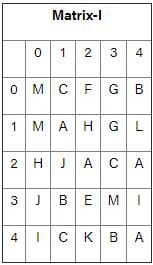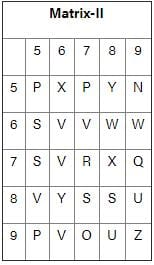Solution:

1 ) 13,86,77,97 → G,Y,R,O

2) 13,57,24,77 → G,P,A,R

3) 10,65,42,79 → M,S,K,Q

4) 20,56,13,75 → H,X,G,S

Hence, 13,86,77,97 represent GYRO.

QUESTION: 4

Arrange the given words in the sequence in which they occur in the dictionary.

1. Triangle
2. Trimmed
3. Tussle
4. Taste
5. Tangle

Solution:

5. Tangle

4. Taste

1. Triangle

2. Trimmed

3. Tussle

QUESTION: 5

In the following question, select the word which cannot be formed using the letters of the given word.

SALVAGING

Solution:

1) SLAIN → SALVAGING → can be formed

2) SLANG → SALVAGING → can be formed

3) GAINS → SALVAGING → can be formed

4) BRINGS → SALVAGING → cannot be formed

Hence, BRINGS cannot be formed from the letters of the given word.

QUESTION: 6

From the given alternatives, select the word which CANNOT be formed using the letters of the given word.

Benevolent

Solution:

Nevin cannot be formed using ‘Benevolent’ because there is no ‘I’ in it.

QUESTION: 7

The prefix which gives a meaningful word when added to the word NATIONAL is?

Solution:

The addition of prefix TRANS to NATIONAL gives the word TRANSNATIONAL which means extending or operating across national boundaries.

QUESTION: 8

In the following question, a series is given. Choose the correct alternative from the given options.

1. Lieutenant Colonel, 2. Captain, 3.  Field Marshal, 4. Major General

Solution:

Given series is a hierarchy of ranks under Indian Army (from top to bottom).

3.Field Marshal > 4. Major General > 1. Lieutenant Colonel > 2. Captain

Hence, the correct sequence is '3412'.

QUESTION: 9

Name a single letter that can be suffixed to the words to form new words?

Lone, Quiet, Sweet, Bright

Solution:

The word that can be suffixed is ‘ly’ to make new words.

Lone + ly = Lonely

Quiet + ly = Quietly

Sweet + ly = Sweetly

Bright + ly = Brightly

Hence, ‘ly’ is the correct alternatively.

QUESTION: 10

Directions: In the question below which one of the given responses would be a meaningful order of the following words in ascending order?

Garnish, cook, purchase, serve, wash

Solution:

Above given is the process of cooking food. The first food is PURCHASED then it is WASHED then it  is COOKED then it GARNISHED and finally it is SERVED to be eaten
Hence, 3, 5, 2, 1, 4 is correct option.

QUESTION: 11

A word is represented by only one set of numbers as given in any one of the alternatives. The sets of numbers given in the alternatives are represented by two classes of alphabets as shown in the given two matrices. The columns and rows of Matrix-I are numbered from 0 to 4 and that of Matrix-II are numbered from 5 to 9. A letter from these matrices can be represented first by its row and next by its column, for example, ‘C’ can be represented by 01, 85, etc., and 'U’ can be represented by 20, 97, etc. Similarly, you have to identify the set for the word “GEEKY”.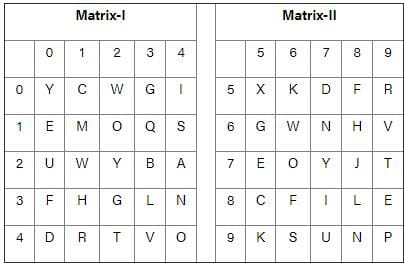Solution:

According to the given information:

1) 32, 01, 89, 56, 22 → G, C, E, K, Y

2) 03, 89, 10, 33, 32 → G, E, E, L, G

3) 65, 10, 75, 95, 00 → G, E, E, K, Y

4) 58, 75, 59, 56, 77 → F, E, R, K, Y

Hence, (65, 10, 75, 95, 00) is the correct answer.

QUESTION: 12

In each of the following questions, which one of the given responses would be a meaningful order of the following words in ascending order?

(a) Uranus
(b) Mercury
(c) Saturn
(d) Earth

Solution:

The correct sequence of planets according to the solar system will be

Mercury, Earth, Saturn, Uranus

Hence the correct sequence will be b, d, c, a

QUESTION: 13

Which one of the given responses would be a meaningful order of the following words?

a. Evaluation
b. Presentation
c. Recap
d. Aim announcement
e. Motivation

Solution:

Given words represent steps followed in the accomplishment of any work.

First of all, MOTIVATION is needed to start any work.

Then AIM is announced which sets the target.

Then PRESENTATION is given which sets the steps to be followed.

Then RECAP is done and then finally EVALUATION is done.

Motivation → Aim announcement → Presentation → Recap → Evaluation.

Hence correct order is e, d, b, c, a.

QUESTION: 14

On arranging Atomic Age, Metallic Age, Stone Age, Alloy Age in a meaningful order (starting from the earliest) which would appear in the 3rd position?

Solution:

The correct order is Stone Age < Metallic Age < Alloy Age < Atomic Age.Hence, Alloy Age would appear in 3rd position.

QUESTION: 15

Directions: In question, which one of the given responses would be a meaningful order of the following?

1)  Gold
2)  Ornaments
3)  Ore
4)  Mine
5)  Process

Solution:

A mine has ore in it, the ore is processed to obtain gold from it. The gold is further used to make ornaments.

Hence, 4 – 3 – 5 – 1 – 2 is the correct sequence.

QUESTION: 16

Arrange the following words in a meaningful order.

1. Key
2. Door
3. Lock
4. Room
5. Switch On

Solution:

Clearly, the logical order is

Key à Lock à Door à Room à Switch on

Hence, 1, 3, 2, 4, 5 is a meaningful order.

QUESTION: 17

Arrange the following words according to a dictionary?

1. Inventory
2. Involuntary
3. Invisible
4. Invariable
5. Investigate

Solution:

The arrangement is as follows:

Invariable → Inventory → Investigate → Invisible → Involuntary

Thus, 4, 1, 5, 3, 2 is the answer.

QUESTION: 18

In the following question which one of the given responses would be a meaningful order of the following?

1. Birth
2. Death
3. Childhood
4. Infancy
7. Old age

Solution:

The correct order is as follows:

Birth → Infancy → Childhood → Adolescence → Adulthood → Old age → Death

Thus the correct order is 1, 4, 3, 5, 6, 7, 2.

QUESTION: 19

Directions: Which one of the given responses would be a meaningful descending order of the following?

1. Major
2. Captain
3. Colonel
5. Lt. General

Solution:

The given options are ranks of officials in the Indian Army. Based on that the descending order is:

Lt. General → Brigadier → Colonel → Major → Captain.

Thus, 5, 4, 3, 1, 2 is the answer.

QUESTION: 20

Arrange the following in the meaningful/logical order:

1. Exhaust
2. Night
3. Day
4. Sleep
5. Work

Solution:

The correct sequence is:

Day → Work → Exhaust → Night → Sleep

As when a day starts one does work for the whole day and due to work one gets exhausted than after day night comes and one goes to sleep to get relief from exhaust situation.

Thus 3, 5, 1, 2, 4 is the answer.

QUESTION: 21

Directions: In a question which one of the given responses would be a meaningful order of the following words?

1. Independence Day
2. Republic Day
3. Gandhiji’s Birthday
4. Pandit Nehruji's Birthday

Solution: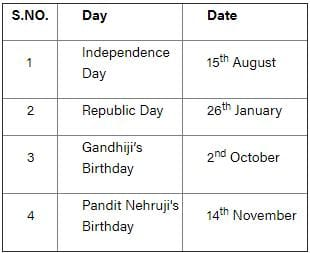Arranging serial numbers according to dates,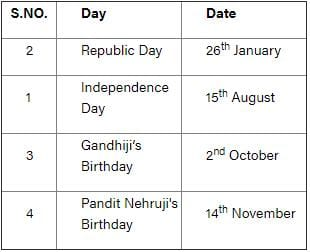New order becomes 2,1,3,4.

QUESTION: 22

Which one of the given responses would be a meaningful order of the following?

1. Substance
2. Atom
3. Molecule
4. Proton

Solution:

We can arrange the options according to their size. Proton has smallest size and substance has largest size.

(4) Proton > (2) Atom > (3) Molecule > (1)Substance

So, the meaningful order is 4, 2, 3, 1.

QUESTION: 23

Arrange the following words according to the dictionary:
(a) Trinity  (b) Tool  (c) Town    (d) Twist   (e)Type

Solution:

(a) Trinity  (b) Tool  (c) Town    (d) Twist   (e)Type

Dictionary Order:

(b) Tool  (c) Town    (a) Trinity    (d) Twist   (e)Type

So, the correct order is b, c, a, d, e.

QUESTION: 24

Which of the following and come in the second number, when they are arranged alphabetically as per in a dictionary.

Solution:

Clearly, the arranged set is as follows:

Passband à Passbook à Passerby à Passible

Here, the second word is Passbook.

QUESTION: 25

Which word will appear third in the dictionary?

Solution:

We will arrange these words according to the dictionary.

1) Meditate

2) Meditation

3) Meditator

4) Mediumism

Hence, “Meditator” comes at third place.

QUESTION: 26

Arrange the following words as per order in the dictionary

1. Dyke
2. Dwindle
3. Dwell
4. Dye

Solution:

Arranging the given words as per the dictionary order,

3. Dwell

2. Dwindle

4. Dye

1. Dyke

Hence the correct dictionary order is 3, 2, 4, 1.

QUESTION: 27

Arrange the following words according to the dictionary.

1. Horse
2. Harness
3. Horizon
4. Horlicks
5. Hormone

Solution:

after arranging as per the order of dictionary we get;

Harness, Horizon, Horlicks, Hormone, Horse

Hence the correct sequence is  2, 3, 4, 5, 1

QUESTION: 28

If the given words are arranged according to the English dictionary, which word will be in third place?

KNOW, KNACK, KNIT, KNOB

Solution:

In dictionary order, the words will be arranged as:

KNACK

KNIT

KNOB

KNOW

We can see that the third word is KNOB.

QUESTION: 29

Which will appear third in the dictionary?

Solution:

In dictionary order, the words are placed in alphabetical order of the first letter which differs between the words.
Therefore, the order will be
Parrot, Partner, Pattern, Practice.
The third word is therefore pattern.

QUESTION: 30

Arrange the following in dictionary order. Then which will be the second word?

1) Specific
2) Spectacle
3) Specified
4) Spectacular

Solution:

In dictionary order, words are put in alphabetically according to the first letter in which they differ.

Thus, the order will be:

Specifi(1), Specified (3), Spectacle (2), Spectacular (4)

QUESTION: 31

In the dictionary, which word comes fourth in arrangement?

Solution:

In dictionary order, the words are placed in alphabetical order of the first letter which differs between the words.

Therefore, the order will be

Spike, Spill, Spine, Split

Therefore, Split is the fourth in the arrangement.

QUESTION: 32

Arrange the following words as per order in the dictionary.

1. Oppose
2. Opposite
3. Opposition
4. Optional
5. Opportune

Solution:

In dictionary order, words are placed in alphabetical order on the basis of the first letter in which they differ. Therefore, the order will be:
Opportune(5), Oppose (1), Opposite (2), Opposition (3), Optional (4).

QUESTION: 33

Which of the following will come forth in the English dictionary?

Solution:

As A comes in the dictionary before E. So word starting with BA are followed by the one starting with BE.

The first word in the alphabet dictionary will be BARK.

The second word will be BARREN.

The third word will be BARROW.

The fourth word will be BELCH.

QUESTION: 34

Direction: Which of the given responses would be a dictionary order of the following?

1) Prescription
2) Prescriber
3) Prescutum
4) Prescript

Solution:

The given words can be arranged according to the dictionary order as follows:

2) Prescriber

4) Prescript

1) Prescription

3) Prescutum

So, the order will be: (2), (4), (1), (3)

QUESTION: 35

Which of the following will come third in the English dictionary?

Solution:

The first word in the dictionary will be RAIN.

The second word in the dictionary will be RAINBOW as the first four letters are same and then letter B comes which comes next in the dictionary series.

The third word in the dictionary will be READas E from the word read comes next in the series as compared to A in the word RAINBOW.

So, READ is the third word which comes in theEnglish dictionary.

QUESTION: 36

Arrange the following words as per order in the dictionary.

I. Silt
II. Silicon
III. Silicate
IV. Silken

Solution:

Arranging them in dictionary order

III. Silicate

II. Silicon

IV. Silken

I. Silt

Hence, III → II → IV → I is correct dictionary order.

QUESTION: 37

Directions: Arrange the following words as per order in the dictionary.

1) Complicate
2) Complicity
3) Complication
4) Compliant

Solution:

According to English Dictionary arrangement will be

COMPLIANT

COMPLICATE

COMPLICATION

COMPLICITY

QUESTION: 38

Arrange the following words as per order in the dictionary and choose the one that comes first.

1. Temple 2. Tenant 3. Terminate 4. Temperature

Solution:

The dictionary order of words is as follows:

4. Temperature,

1. Temple,

2. Tenant,

3. Terminate

Hence the first word in the order is Temperature.

QUESTION: 39

Arrange the following words according to English Dictionary.

(a) abase
(b) absurd
(c) abysmal
(d) abash

Solution:

After arranging as per the English dictionary:

Abase, abash, absurd, abysmal

Hence a, d, b, c is the correct option.

QUESTION: 40

Which single letter can be prefixed to the following words in order to obtain entirely new words? (the Same letter has to be prefixed in all the five words of each)

EAT   OUR  IS     AS    AT

Solution: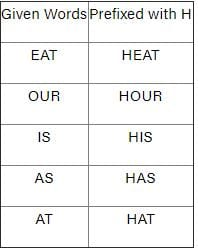Clearly, if the words are prefixed with H, meaningful words can be formed, otherwise, with the other letters, the words are meaningless.

QUESTION: 41

Name a single letter, which can be prefixed to the following words in order to obtain an entirely new word?

TILL   TABLE PILE  TAB   PRING

Solution:

Let’s check for each option,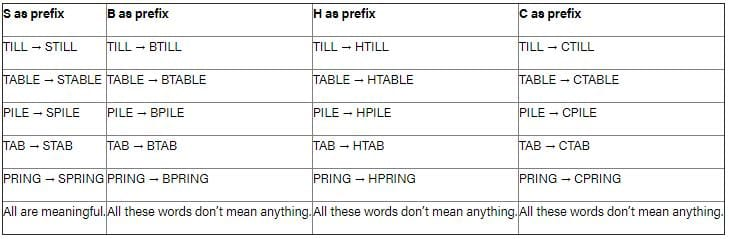Hence, S is the correct answer.

QUESTION: 42

Name a single letter that can be suffixed to the following words to form new words?

HAT BAR BAT PIN BATH

Solution: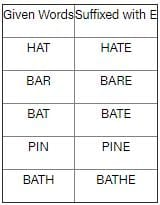Clearly, if the words are suffixed with E, meaningful words can be formed, otherwise, with the other letters, the words are meaningless.

Hence, E is the correct answer.

QUESTION: 43

Which of the following Jumbled words isn’t an animal or an Insect?

Solution:

Let’s unjumbled the given options:
A. LATHPEEN ⇒ Elephant
B. TICRECK ⇒ CRICKET
C. FEFEOC ⇒ COFFEE ⇒ Not an animal but a beverage.
D. TAR ⇒ RAT
Clearly, Coffee i.e. FEFEOC is the odd-one out.

QUESTION: 44

Directions: In the following question, select the one which is different from the other three alternatives.

Solution:
• SNINET is an anagram for TENNIS
• LABLOTOF is an anagram for FOOTBALL
• CEKTRIC is an anagram for CRICKET.
• HEFRODA is an anagram for FORHEAD.

Since the 1st three are sports, hence SCHAMOT is the odd one out.

QUESTION: 45

Rearrange the jumbled up letters in their natural sequences and find the odd one out.

Solution:

Let’s unjumbled the given options:
A. LATHPEEN ⇒ Elephant
B. TICRECK ⇒ CRICKET.
FEFEOC ⇒ COFFEE ⇒ Not an animal but a beverage.
D. TAR ⇒ RAT
Clearly, Coffee i.e. FEFEOC is the odd-one out.

QUESTION: 46

In the following letters, how many times does ‘P’ occur in such a way that immediately after ‘P’, N O should occur?

A P N Q P N O S P T O Z P N O Y M P N O

Solution:

A P N Q N O S P T O Z N O Y M N O

We can clearly see that there are 3 P after which N O occurs.

So, the correct answer is 3.

QUESTION: 47

Directions: In question, some letters are given with numbers from 1 to 9 or 6. Select the sequence of numbers which arranges the letters into a meaningful word.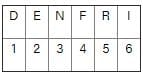Solution:

Let’s check each option,

1) 4 5 6 2 3 1 → FRIEND which is meaningful.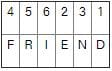2) 3 2 1 6 5 4 → Not meaningful.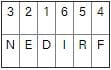3) 6 4 2 3 5 1 → Not meaningful.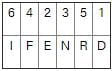4) 1 2 5 4 6 3 → not meaningful.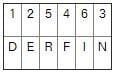Hence answer is 4 5 6 2 3 1.

QUESTION: 48

A word is represented by only one set of numbers as given in any one of the alternatives. The sets of numbers given in the alternatives are represented by two classes of alphabets as in the two matrices given below. The columns and rows of the matrix I am numbered from 0 to 4 and that of Matrix II are numbered from 5 to 9. A letter from these matrices can be represented first by its row and next by its column, e.g., ‘U’ can be represented by 01, 33, etc., and ‘W’ can be represented by 55, 78, etc., similarly, you have to identify the set for the word ‘LEARN’.

Matrix I                         Matrix II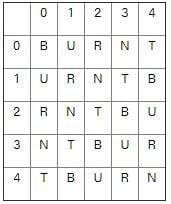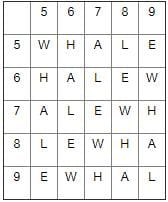Solution:

Let’s check all option,

A. 76, 95, 66, 02, 21 → LEARN

B. 76, 95, 66, 01, 21 → LEAUN

C. 66, 95, 67, 02, 21 → AELRN

D. 76, 85, 66, 02, 11 → LLARR

Thus the correct option is 76, 95, 66, 02, 21.

QUESTION: 49

In this question, the sets of letters given in the alternatives are represented. The columns and rows of Matrix are numbered from J to N. A number from these matrices can be represented first by its row and next by its column, e.g., ‘1’ can be represented by EA, BC etc., and '2' can be represented by LM, NM, etc. Similarly you have to identify the set for the letter for number ‘4321’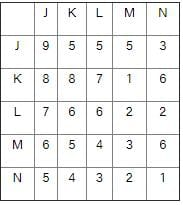Solution:

1) ML, MM, NM, NN ⇒ 4, 3, 2, 1

2) NK, NL, KM, NN ⇒ 4, 3, 1, 1

3) ML, MM, LN, LM ⇒ 4, 3, 2, 2

4) NK, LN, LM, KM ⇒ 4, 2, 2, 1

Therefore, “ML, MM, NM, NN” are set of letter which represent word “4321”

QUESTION: 50

In this question, the sets of letters given in the alternatives are represented. The columns and rows of Matrix are numbered from J to N. A number from these matrices can be represented first by its row and next by its column, e.g., ‘1’ can be represented by EA, BC etc., and '2' can be represented by LM, NM, etc. Similarly, you have to identify the set for the letter for number ‘3579’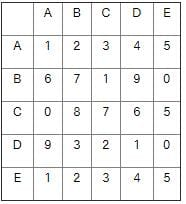Solution:

1) DB, AE, BB, AD ⇒ 3, 5, 7, 4

2) DB, BE, BB, BD ⇒ 3, 0, 7, 9

3) DB, AE, BC, BD ⇒ 3, 5, 1, 9

4) DB, AE, BB, BD ⇒ 3, 5, 7, 9

Therefore, “DB, AE, BB, BD” are set of letter which represent word “3579”

QUESTION: 51

In this question, the sets of the letter given in the alternatives are represented. The columns and rows of Matrix I are numbered from A to E. A number from these matrices can be represented first by its row and next by its column, e.g., ‘1’ can be represented by EA, BC, etc., and '2' can be represented by LM, NM, etc. Similarly, you have to identify the set for the letter for number ‘97632’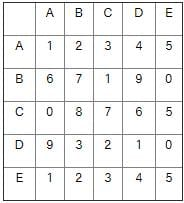Solution:

1) DA, CB, BB, DD, EB ⇒ 9, 8, 7, 1, 2

2) BD, CC, CD, AC, AB  9, 7, 6, 3, 2

3) DA, BC, BB, DD, EB ⇒ 9, 1, 7, 1, 2

4) BD, CC, CD, CA, AB ⇒ 9, 7, 6, 1, 2

Therefore, “BD, CC, CD, AC, AB” are set of letter which represent word “97632”

QUESTION: 52

In this question, the sets of numbers given in the alternatives are represented. The columns and rows of Matrix I are numbered from 1 to 5 and that of Matrix II are numbered from 5 to 9. A letter from these matrices can be represented first by its row and next by its column, e.g., ‘M’ can be represented by 12, 25, etc., and 'P' can be represented by 67, 58, etc. Similarly, you have to identify the set for the word ‘NOBAL’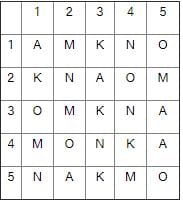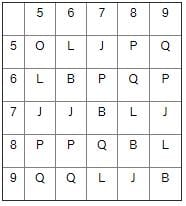Solution:

1) 22, 24, 76, 52, 89 ⇒ N, O, J, A, L

2) 43, 24, 88, 34, 56 ⇒ N, O, B, N, L

3) 14, 55, 66, 11, 78 ⇒ N, O, B, A, L

4) 34, 15, 77, 35, 79 ⇒ N, O, B, A, J

Therefore, “14, 55, 66, 11, 78” are set of letter which represent word “NOBAL”

QUESTION: 53

In this question, the sets of numbers given in the alternatives are represented. The columns and rows of Matrix I are numbered from 1 to 5 and that of Matrix II are numbered from 5 to 9. A letter from these matrices can be represented first by its row and next by its column, e.g., ‘M’ can be represented by 12, 25, etc., and 'P' can be represented by 67, 58, etc. Similarly, you have to identify the set for the word ‘PLANK’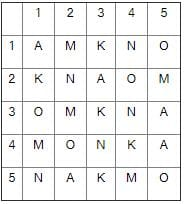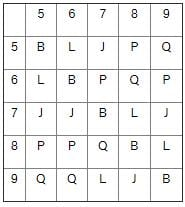Solution:

1) 58, 56, 11, 15, 44 ⇒ P, L, A, O, K

2) 67, 65, 22, 11, 33 ⇒ P, L, N, A, K

3) 85, 78, 23, 43, 42 ⇒ P, L, A, N, O

4) 86, 97, 45, 51, 53 ⇒ P, L, A, N, K

Therefore, “88, 97, 45, 51, 53” are set of letter which represent word “PLANK”

QUESTION: 54

In this question, the sets of numbers given in the alternatives are represented. The columns and rows of Matrix I are numbered from 1 to 5 and that of Matrix II are numbered from 5 to 9. A letter from these matrices can be represented first by its row and next by its column, e.g., ‘M’ can be represented by 12, 25 etc., and 'P' can be represented by 67, 58, etc. Similarly you have to identify the set for the word ‘BLANK’Solution:

1) 55, 56, 11, 15, 44 ⇒ B, L, A, O, K

2) 66, 65, 22, 11, 33  B, L, N, A, K

3) 77, 78, 23, 43, 42 ⇒ B, L, A, N, O

4) 88, 97, 45, 51, 53 ⇒ B, L, A, N, K

Therefore, “88, 97, 45, 51, 53” are set of letter which represent word “BLANK”

QUESTION: 55

In this question, the sets of numbers given in the alternatives are represented. The columns and rows of Matrix I are numbered from 1 to 5 and that of Matrix II are numbered from 5 to 9. A letter from these matrices can be represented first by its row and next by its column, e.g., ‘N’ can be represented by 14, 34, etc., and 'J' can be represented by 76, 57, etc. Similarly, you have to identify the set for the word ‘PAJAMA’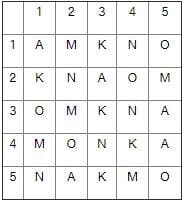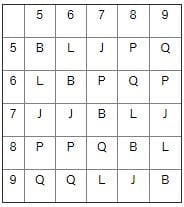Solution:

1) 85, 35, 56, 45, 32, 25 ⇒ P, A, J, A, M, M

2) 86, 45, 57, 35, 32, 34  P, A, J, A, M, N

3) 67, 23, 79, 45, 25, 45 ⇒ P, A, J, A, M, A

4) 58, 14, 57, 45, 32, 23 ⇒ P, N, J, A, M, A

Therefore, “67, 23, 79, 45, 25, 45” are set of letter which represent word “PAJAMA”

QUESTION: 56

In this question, the sets of numbers given in the alternatives are represented. The columns and rows of Matrix I are numbered from 1 to 4 and that of Matrix II are numbered from 5 to 8. A letter from these matrices can be represented first by its row and next by its column, e.g., ‘N’ can be represented by 33, 57, etc., and 'K' can be represented by 21, 56, etc. Similarly you have to identify the set for the word ‘SHNSE’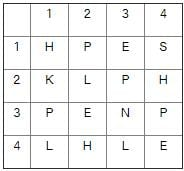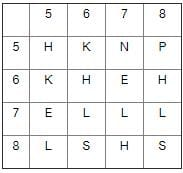Solution:

1) 14, 13, 57, 13, 88 ⇒ S, E, N, E, S

2) 86, 42, 33, 14, 44 ⇒ S, H, N, S, E

3) 14, 67, 33, 86, 44 ⇒ S, E, N, S, E

4) 86, 32, 33, 13, 88 ⇒ S, E, N, E, S

Therefore, “86, 42, 33, 14, 44” are set of letter which represent word “SHNSE”

QUESTION: 57

In this question, the sets of numbers given in the alternatives are represented. The columns and rows of Matrix I are numbered from 1 to 4 and that of Matrix II are numbered from 5 to 8. A letter from these matrices can be represented first by its row and next by its column, e.g., ‘N’ can be represented by 33, 57, etc., and 'K' can be represented by 21, 56, etc. Similarly you have to identify the set for the word ‘SENSE’Solution:

1) 14, 13, 57, 13, 88 ⇒ S, E, N, E, S

2) 86, 42, 33, 14, 44 ⇒ S, H, N, S, E

3) 14, 67, 33, 86, 44 ⇒ S, E, N, S, E

4) 86, 32, 33, 13, 88 ⇒ S, E, N, E, S

Therefore, “14, 67, 33, 86, 44” are set of letter which represent word “SENSE”

QUESTION: 58

A word is represented by only one set of numbers as given in any one of the alternatives. The sets of numbers given in the alternatives are represented by two classes of alphabets as in two matrices given below. The columns and rows of Matrix I are numbered from 0 to 4 and that of Matrix II are numbered from 5 to 9. A letter from these matrices can be represented first by its row and next by its column. e.g., ‘I’ can be represented by 03, 22, etc. and ‘R’ can be represented by 57, 68, etc. Similarly, you have to identify the set for the word ‘BALD’.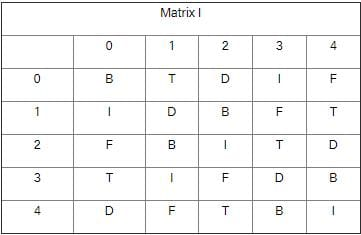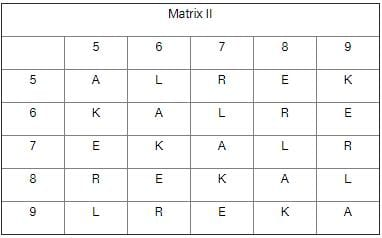Solution:

a) 12, 99, 65, 24 = BAKD

b) 21, 88, 95, 24 = BALD

c) 43, 55, 67, 04 = BALF

d) 34, 77, 76, 42 = BAKT

Hence, 21, 88, 95, 24 = BALD is correct option.

QUESTION: 59

A word is represented by only one set of numbers us given in any one of the alternatives. The sets of numbers given in the alternatives are represented by two classes of alphabets as in two matrices given below, The columns and rows of Matrix I are numbered from 0 to 4 and that of Matrix ll are numbered from 5 to 9. A letter from these matrices can be represented first by its row and next by its column. e.g,, I can be represented by 03. 22; etc.,and ‘R‘ can be represented by 57, 68 etc. Similarly, you have to identify the set for the word `FIRE’.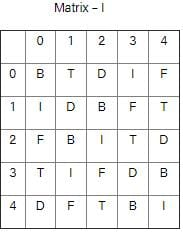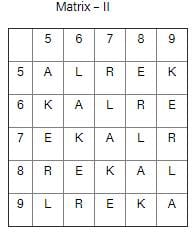Solution:

20 → F, 22 → I, 96 → R, 75 → E = FIRE.

a) 12, 24, 99, 65 → D, D, A, K → DDAK

b) 20, 22, 96, 75 → F, I, R, E → “FIRE”

c) 41, 03, 55, 67 → F, I, A, L → FIAL

d) 34, 42, 78, 79 → B, T, L, R → BTLR

Hence, “FIRE” word is formed with 20, 22, 96, 75.

QUESTION: 60

In the following question, a word is represented by only one set of numbers as given in any one of the alternatives. The set of numbers given in the alternatives are represented by two classes of alphabets as in two matrices given below. The columns and rows of Matrix I are numbered from 0 to 4 and that of Matrix II are numbered from 5 to 9. A letter from these matrices can be represented first by its row and next by its column, e.g., ‘F’ can be represented by 14, 21, etc. and ‘O’ can be represented by 67, 98, etc. Identify the set for the word TEAR.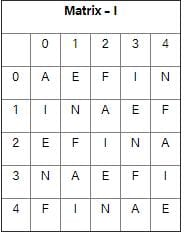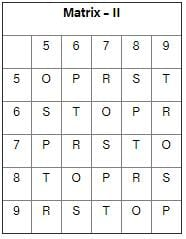Solution:

1. 21, 22, 88, 33 = FIRF

2. 14, 10, 69, 14 = FIRF

3. 66, 20, 43, 57 = TEAR

4. 02, 03, 57, 01 = FIRE

Hence, “66, 20, 43, 57 = TEAR” is a correct option.

QUESTION: 61

A word is represented by only one set of numbers as given in any one of the alternatives. The sets of numbers given in the alternatives are represented by two classes of alphabets as in two matrices given below. The columns and rows of Matrix ZI are numbered from 0 to 4 and that of Matrix II are numbered from 5 to 9. A letter from these matrices can be represented first by its row and next by its column, e.g., ‘G’ can be represented by 02, 14, etc., and ‘P’ can be represented by 66, 78 etc. similarly, you have to identify the set for the word ‘FINE’.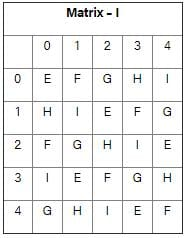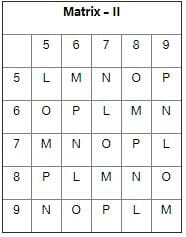Solution:

1. 20, 11, 69, 43 = FINE

2. 01, 11, 69, 40 = FING

3. 13, 31, 76, 42 = FENI

4. 32, 11, 68, 42 = FIMI

Hence 20, 11, 69, 43 is correct option

QUESTION: 62

In the following question, a word is represented by a set of numbers as given in any one of the alternatives. The sets of numbers given in the alternatives are represented by alphabets as in the matrices given below. The columns and rows of the matrix are numbered from 1 to 6. A letter from these matrices can be represented first by its row and next by its column number, e.g., ‘A’ can be represented by 42, ‘S’ can be represented by 21, etc. Similarly, you have to identify the set for the word ‘PLAY’.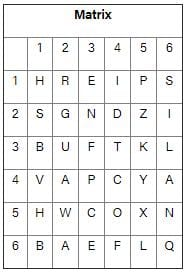Solution:

1) 43, 36, 42, 23 → PLAN → Wrong

2) 43, 32, 33, 33 → PUFF → Wrong

3) 15, 12, 42, 45 → PRAY→ Wrong

4) 43, 65, 62, 45 → PLAY → Right

Hence correct answer is 43, 65, 62, 45.

QUESTION: 63

A word is represented by only one set of numbers as given in any one of the alternatives. The sets of numbers given in the alternatives are represented by two classes of alphabets as in two matrices given below. The columns and rows of matrix I are numbered from 0 to 4 and that of Matrix II are numbered from 5 to 9. A letter from these matrices can be represented first by its row and next by its column, e.g., ‘N’ can be represented by 02, 20, etc. and ‘Q’ can be represented by 56, 78 etc. similarly, you have to identify the set for the word ‘POSTOR’.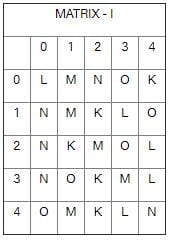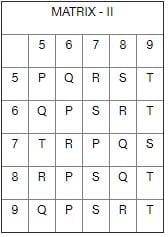Solution:

A) 66, 03, 58, 57, 03, 76 → POSROR

B) 77, 79, 67, 59, 14, 57 → PSSTOR

C) 86, 23, 79, 59, 89, 76 → POSTTR

D) 96, 31, 97, 59, 40, 98 → POSTOR

Thus code for POSTOR from given options is 96, 31, 97, 59, 40, 98.

QUESTION: 64

In this question, the sets of numbers given in the alternatives are represented. The columns and rows of Matrix I are numbered from 0 to 4 and that of Matrix II are numbered from 5 to 9. A letter from these matrices can be represented first by its row and next by its column, e.g., O can be represented by 03, 14, etc., and 'K' can be represented by 56, 65, etc. Similarly you have to identify the set for the word 'EASE'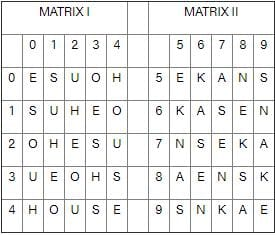Solution:

Let’s check each option,

1) 55, 85, 44, 42 → EAEU

2) 77, 85, 88, 44 → EASE

3) 77, 66, 31, 44 → EAEE

4) 00, 98, 23, 98 → EASA

Thus the correct option is “77, 85, 88, 44”.

QUESTION: 65

In the question, a word is represented by only one set of numbers as given in any one of the alternatives. The sets of numbers given in the alternatives are represented by two classes of alphabets as in two matrices given below. The columns and rows of Matrix I are numbered from 0 to 4 and that of Matrix II are numbered from 5 to 9. A letter from these matrices can be represented first by its row and next by its column, e.g., 'G' can be represented by 04, 40 etc., and 'K' can be represented by 56, 75 etc.

Similarly, you have to identify the set for the word 'NILE'.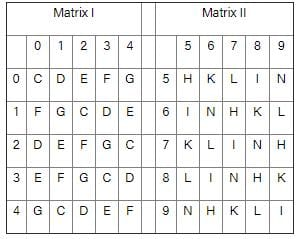Solution:

The given options represent following words,

1) 56, 58, 03, 02 → KIFE

2) 59, 77, 85, 43 → NILE

3) 56, 62, 03, 02 → 62 is invalid number

4) 57, 60, 10, 02 → 60 is invalid number

Hence the correct code for NILE is 59, 77, 85, 43.

QUESTION: 66

A word is represented by only one set of numbers as given in any one of the alternatives. The sets of numbers given in the alternatives are represented by two classes of alphabets as in two matrices given below. The columns and rows of Matrix-II are numbered from 5 to 9. A letter from these matrices can be represented first by its row and next by its column. e.g., D can be represented by 02, 10, etc., and 'R' can be represented by 55, 67, etc. Similarly you have to identify the set for the word 'BEAR'.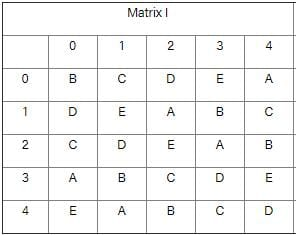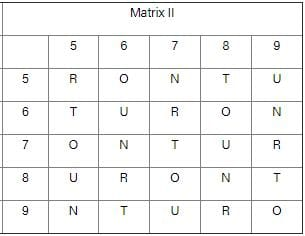Solution:

The given options represent following words,

1) 13, 11, 12, 78 → BEAU

2) 31, 22, 23, 97 → BEAU

3) 42, 34, 42, 79 → BEBR

4) 24, 40, 23, 67 → BEAR

Hence, option 4 is the correct set to represent the word ‘BEAR’.

QUESTION: 67

From the given alternatives, select the word which cannot be formed using the letters of the given word.

BANGALORE

Solution:

Let’s check each option,

1) GARBAGE → Cannot be formed as there is only 1 ‘G’ in BANGALORE.

2) ORANGE → Can be formed as BANGALORE.

3) LARGE → Can be formed as BANGALORE.

4) BANGLE → Can be formed as BANGALORE.

Hence word GARBAGE cannot be formed from word BANGALORE.

QUESTION: 68

From the given alternatives, select the word which can be formed using the letters given in the word:

ABOMINABLE

Solution:

Let’s check each option,

1) BOWEL → Cannot be formed as there is no ‘W’ in ABOMINABLE.

2) METAL → Cannot be formed as there is no ‘T’ in ABOMINABLE.

3) BLAND → Cannot be formed as there is no ‘D’ in ABOMINABLE.

4) BANAL→ Can be formed as ABOMINABLE.

Hence only word BANAL can be formed from the word ABOMINABLE.

QUESTION: 69

From the given alternative words, select the one which can be formed using the letters of the given word.

PRAGMATIC

Solution:

Let’s check each option,

1) GUITAR → cannot be formed as there is no ‘U’ in PRAGMATIC.

2) AGMARK → cannot be formed as there is no ‘K’ in PRAGMATIC.

3) GAME → cannot be formed as there is no ‘E’ in PRAGMATIC.

4) MAGIC → can be formed as PRAGMATIC.

Prudently, MAGIC contains all the letters of the word PRAGMATIC.

QUESTION: 70

From the given alternatives words, select the word which can be formed using the letters of the given word:

‘DETERMINATION’

Solution:

Let’s check each option,

1) DECLARATION → cannot be formed as there is no ‘C’ and 'L' and only 1 'A' in ‘DETERMINATION’.

2) NATIONAL → cannot be formed as there is only 1 ‘A’ and no 'L' in ‘DETERMINATION’.

3) TERMINATED → can be formed as DETERMINATION.

4) DEVIATION → cannot be formed as there is no ‘V’ in ‘DETERMINATION’.

Hence only word TERMINATED can be formed from word DETERMINATION.

QUESTION: 71

From the given alternatives words, select the one which can be formed using the letters of the given word

IMMEDIATELY

Solution:

Here, LIMITED is the only word which can be formed from the above word as in other options like,

DIALECT → No ‘C’ in IMMEDIATELY.

DIAMETER → No ‘R’ in IMMEDIATELY.

DICTATE → No ‘C’ in IMMEDIATELY.

Hence only word LIMITED can be formed.

QUESTION: 72

Some letters are given with numbers from 2 to 9. Select the sequence of numbers which arranges the letters into a meaningful word.

N   A    E    X   I    M   O   T    N     A     I

9    2    3    4   5   6     7   8    9     2     5

Solution:

Let us check each option one by one: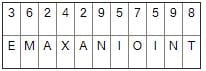This word is not a meaningful word.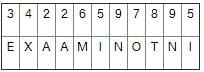This word is not a meaningful word.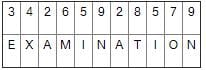This is a meaningful word.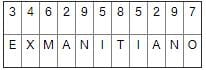This is not a meaningful word.

Thus by sequence 3,4,2,6,5,9,2,8,5,7,9 a meaningful word i.e. EXAMINATION is formed.

QUESTION: 73

From the given alternatives words, select the word which can be formed using the letters of the given word.

IMMEDIATELY

Solution:
1. DIALECT – ‘C’ is not present in IMMEDIATELY.
2. LIMITED – Can be formed.
3. DIAMETER – ‘R’ is not present in IMMEDIATELY
4. DICTATE – ‘C’ is not present in IMMEDIATELY. Also we need 2 ‘T’s.

QUESTION: 74

From the given alternative words, select the word which can be formed using the letters of the given word.

OMNIPRESENT

Solution:
• ERASER – We need 2 ’R’s and an ‘A’ which is not present in OMNIPRESENT.
• TENSION – Can be formed.
• ESCORT – We need a ‘C’ which is not present in OMNIPRESENT.
• PRESIDENT – We need a ‘D’ which is not present in OMNIPRESENT.

Hence only TENSION can be formed.

QUESTION: 75

From the given alternatives select the word which cannot be formed using the letters of the given word :

COMMUNICATION

Solution:

Here, all the words except “UNISON” can be formed as there is no ‘S’ present in the above word.

QUESTION: 76

Select the word which cannot be formed from PERPETUATION.

Solution:

Retention contains two N’s while there is only one in word PERPETUATION.

QUESTION: 77

Directions: From the given alternative words, select the word which cannot be formed using the letters of the given word.

POLYTHEISM

Solution:

Let’s check each option

PISTOL → POLYTHEISM

THESIS → POLYTHEISM, S is missing

SMITH → POLYTHEISM

HOSTEL → POLYTHEISM

Hence word THESIS cannot be formed from the word POLYTHEISM.

QUESTION: 78

From the given alternatives select the word which cannot be formed using the letters of the given word.

‘EXAMINATION’

Solution:

Let’s check each option,

ANIMATION → EXAMINATION

ANIMAL → EXAMINATION, L is missing.

EXAM →EXAMINATION

NATION → EXAMINATION

Hence word ANIMAL cannot be formed using the letters of the word EXAMINATION.

QUESTION: 79

From the given alternatives, select the word which cannot be formed using the letters given in the words.

CHIEF MINISTER

Solution:

Word SISTER contains two ‘S’ but there is only one in CHIEF MINISTER.

Hence word SISTER cannot be formed from the letters of the word CHIEF MINISTER.

QUESTION: 80

Form the given alternatives, find the word which cannot be formed from the letters used in the given word.

GERMINATION

Solution:

Let’s check each option,

1) ORIGINATE → Can be formed as GERMINATION.

2) TERMINAL → Cannot be formed as there is ‘L’ in GERMINATION.

3) EMIGRANT → Can be formed as GERMINATION.

4) GERMAN → Can be formed as GERMINATION.

Thus word TERMINAL cannot be formed from the word GERMINATION.Use Code STAYHOME200 and get INR 200 additional OFF Use Coupon Code